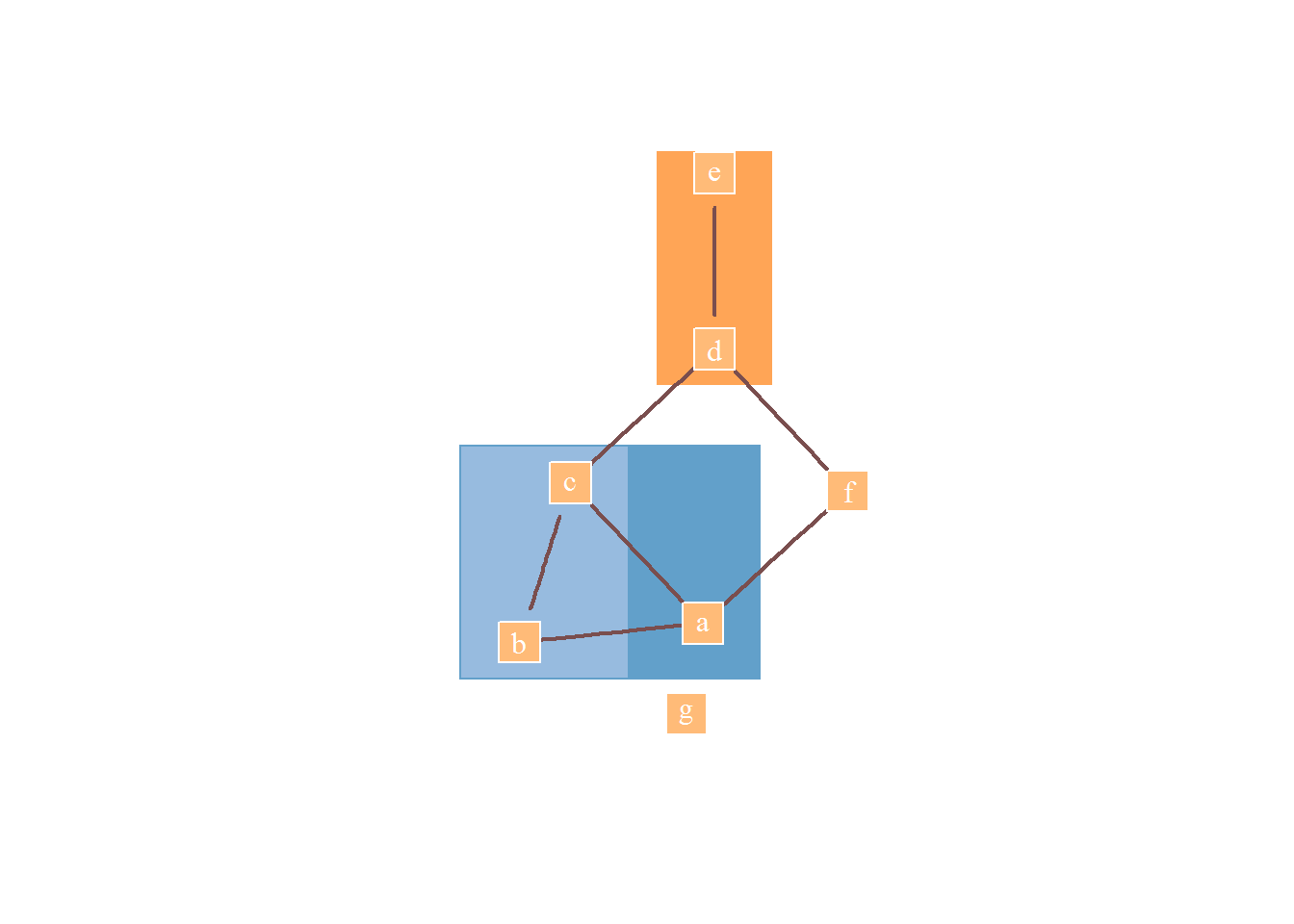Week 46 | colaR

This Week’s Widget - colaR

Tim Dwyer makes really great stuff. As one recent example, see this video preview of HOLA: Human-like Orthogonal Network Layout which won Best Infovis Paper at VIS 2015. Unfortunately though, Dwyer does not make his great stuff in R. This is where what I call super-isomorphism with htmlwidgets and Jeroen Ooms’ V8 becomes very handy. We can get all the benefit of something like Dwyer’s WebCola without the need to rewrite or port code.

I hesitate to call this week’s colaR an htmlwidget, since I only use the htmlwidget infrastructure currently to provide the JavaScript dependencies for HTML or V8. I can’t help dedicating this week to this amazing JavaScript. I hope someone smarter, more creative, and/or more diligent can take this and run with it. Of all the htmlwidgets, this is one I really, really hope someone pushes to its full potential.

Installation

This is not on CRAN, so to install we will need some help from devtools::install_github.

devtools::install_github("timelyportfolio/colaR")

Examples

We’ll explore some super-isomorphism. First, let’s recreate the gridified small group example with igraph and V8 + colaR. I’ll try to get as close as possible to the final output minus the interactivity of course.

igraph and V8

# devtools::install_github("timelyportfolio/colaR")
# demo how we can use WebCola in R with V8

library(V8)
library(jsonlite)
library(igraph)
library(scales)
library(pipeR)

ctx = new_context(global="window")

ctx$source(system.file("htmlwidgets/lib/WebCola/cola.js",package = "colaR")) ### small grouped example group_json <- fromJSON( system.file( "htmlwidgets/lib/WebCola/examples/graphdata/smallgrouped.json", package = "colaR" ) ) # need to get forEach polyfill ctx$source(
"https://cdnjs.cloudflare.com/ajax/libs/es5-shim/4.1.10/es5-shim.min.js"
)

# code to recreate small group example
js_group <- '
// console.assert does not exists
console = {}
console.assert = function(){};

var width = 960,
height = 500

graph = {
"nodes":[
{"name":"a","width":60,"height":40},
{"name":"b","width":60,"height":40},
{"name":"c","width":60,"height":40},
{"name":"d","width":60,"height":40},
{"name":"e","width":60,"height":40},
{"name":"f","width":60,"height":40},
{"name":"g","width":60,"height":40}
],
{"source":1,"target":2},
{"source":2,"target":3},
{"source":3,"target":4},
{"source":0,"target":1},
{"source":2,"target":0},
{"source":3,"target":5},
{"source":0,"target":5}
],
"groups":[
{"leaves":, "groups":},
{"leaves":[1,2]},
{"leaves":[3,4]}
]
}

var g_cola = new cola.Layout()
.avoidOverlaps(true)
.handleDisconnected(false)
.size([width, height]);

g_cola
.nodes(graph.nodes)
.groups(graph.groups)
.start()

'

# run the small group JS code in V8
ctx$eval(js_group) ##  "[object Object]" # make an igraph from the graph JSON igf <- graph.data.frame( group_json$links + 1
,vertices =  data.frame(id = 1:nrow(group_json$nodes),group_json$nodes)
)

# use WebCola in V8 to layout the graph
igf_layout <- ctx$get(' graph.nodes.map(function(d){ return [d.x,-d.y] }) ') # plot the small group as much like the example as possible # start with an empty plot plot.new() # set our x and y scale limits plot.window(xlim=c(-3,3),ylim=c(-1,1)) ctx$get('graph.groups.map(function(d){return d.bounds})') %>>%
(
rect(
xleft = rescale(.$x, c(-1,1), from = range(igf_layout[,1])) ,ybottom = rescale(-.$Y, c(-1,1), range(igf_layout[,2]))
,xright = rescale(.$X, c(-1,1), from = range(igf_layout[,1])) ,ytop = rescale(-.$y, c(-1,1), range(igf_layout[,2]))
,col = c(
rgb(31/255, 119/255, 180/255,alpha=0.7)
,rgb(174/255, 199/255, 232/255,alpha=0.7)
,rgb(255/255, 127/255, 14/255,alpha=0.7)
)
,border = NA
)
)

plot(
igf
, layout = igf_layout
, vertex.shape = "square"
, vertex.label.color = "white"
, vertex.color = rgb(255, 187, 120, maxColorValue = 255)
, vertex.frame.color = "white"
, vertex.size = 25
, edge.arrow.mode = "-"
, edge.color = "#7A4E4E"
, edge.width= 2
#, mark.groups = lapply(group_json$groups$leaves,function(x)x+1)
, add = TRUE
)htmltools

Now let’s use htmltools to recreate the alignment constraints example exactly with full interactivity. Note, I deserve no credit for this code. I’ll include the condensed version below. Please see htmltools_alignment_example.R for the full source.

# devtools::install_github("timelyportfolio/colaR")
library(htmltools)
library(colaR)

# almost all code copied/pasted from
#   WebCola example
#   https://github.com/tgdwyer/WebCola/blob/master/WebCola/examples/alignment.html
#   http://marvl.infotech.monash.edu/webcola/examples/alignment.html
#  make our alignment page
browsable(tagList(
tags$html( tags$head(
webcola_alignment_style()
),
tagsh1("Alignment constraints with guidelines"), webcola_alignment_script(), tagsp("Nodes 'a', 'b' and 'e' are constrained to the same y-coordinate.  'b', 'c' and 'd' are constrained to the same x.")
),
# add d3 and WebCola
d3(),
cola()
))

Thanks

Thanks Tim Dwyer for WebCola and all his research.

As always, thanks to

• Ramnath Vaidyanathan and RStudio for htmlwidgets
• all the contributors to R and JavaScript